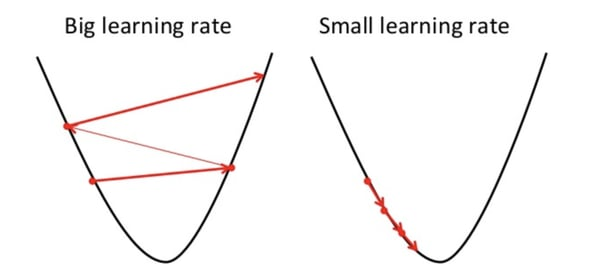Trusted answers to developer questions
Trusted Answers to Developer Questions

Related Tags

learning
rate
machine learning
descent

# Learning Rate in Machine learningEducative Answers Team

Grokking Modern System Design Interview for Engineers & Managers

Ace your System Design Interview and take your career to the next level. Learn to handle the design of applications like Netflix, Quora, Facebook, Uber, and many more in a 45-min interview. Learn the RESHADED framework for architecting web-scale applications by determining requirements, constraints, and assumptions before diving into a step-by-step design process.

The weights of a neural network cannot be calculated using an analytical method. Instead, the weights must be discovered via an empirical optimization procedure called stochastic gradient descent.

Stochastic gradient descent is an optimization algorithm that estimates the error gradient for the current state of the model using examples from the training dataset, and then updates the weights of the model using backpropagation.

Learning rate is a hyper-parameter that controls the weights of our neural network with respect to the loss gradient. It defines how quickly the neural network updates the concepts it has learned.

A desirable learning rate is low enough that the network converges to something useful, but high enough that it can be trained in a reasonable amount of time.A small learning rate makes the model converge slowly to the global minimum loss.

Smaller learning rates require more training epochs (requires more time to train) due to the smaller changes made to the weights in each update, whereas larger learning rates result in rapid changes and require fewer training epochs. ​ However, larger learning rates often result in a sub-optimal final set of weights.

## Adaptive learning rate

Adaptive learning rates allow the training algorithm to monitor the performance of the model and automatically adjust the learning rate for the best performance.

The most basic model of this decreases the learning rate once the performance of the model reaches a plateau. The model does so by decreasing the learning rate by a factor of two or an order of magnitude. However, the learning rate can be increased again if the performance doesn’t improve.

Neural networks with adaptive learning rates usually outperform ones with fixed learning rates.

RELATED TAGS

learning
rate
machine learning
descent